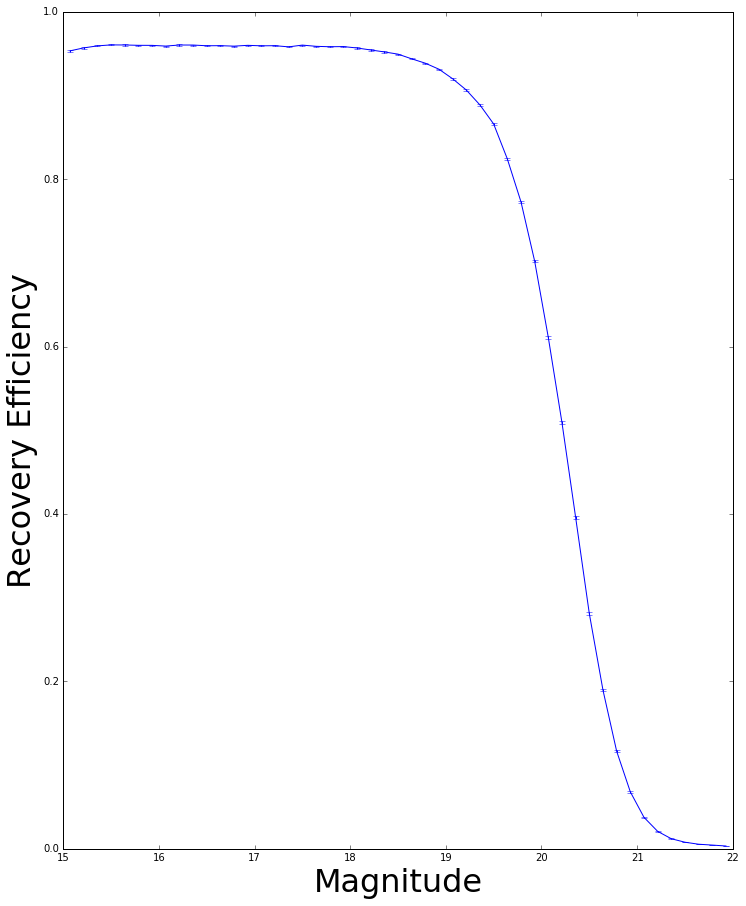# Uncertainties on the Efficiency

Problem: What is the proper way of calculating errors on an efficiency?

We define the efficiency as $\epsilon = \frac{k}{N}$ where N is the number of fakes simualted and k is the number of fakes recovered which pass our selection cuts.

## The Bayesian Method¶

### Primer¶

The probability of recovering $k$ events given a true efficiency, $\epsilon$ with $N$ trials is expressed through the binomial distribution (prior $I$).

\begin{equation} P(k \mid \epsilon, N, I) = \frac{N!}{k!(N-k)!} \epsilon^k(1-\epsilon)^{N-k} \end{equation}

However, we do not know the true efficiency, but we do have our data of k events out of N passing.

What we need to determine instead is

\begin{equation} P(\epsilon \mid k, N, I) \end{equation}

from this also find the most probable value of $\epsilon$ and the confidence interval of $\pm d\epsilon$

We employ Bayes’ Theorem: $P(A \mid BC) \rightarrow P(B \mid AC)$

### Applying Bayes to our problem¶

\begin{equation} P(\epsilon \mid k, N, I) = \frac{P(k \mid \epsilon, N, I)P(\epsilon \mid N, I)}{Z} \end{equation}

Where Z is a normalisation constant. For a derivation of this constant see Paterno 2003 here [http://home.fnal.gov/~paterno/images/effic.pdf]

Following Paterno, we find

\begin{equation} P(\epsilon \mid k, N, I) = \frac{\Gamma(N+2)}{\Gamma(k+1)\Gamma(N-k+1)}\epsilon^k(1-\epsilon)^{N+k} \end{equation}

Note: Finding the peak of this funciton (take the derivative, equal it to 0, solve. It’s long I did it, wouldn’t reccommend!) you will get the true efficiency. You will notice that is equals $\frac{k}{N}$. Great, that’s what we expected!

$P(\epsilon \mid k, N, I)$ is not a delta function and will have some associated uncertainty. We will quote all uncertainties as the 1$\sigma$ uncertainty, i.e. 0.683

Therefore we want to minimise $\beta - \alpha$ subject to the constraint

\begin{equation} \int_{\alpha}^{\beta}P(\epsilon \mid k, N, I) = 0.683 \end{equation}

#### CALCEFF¶

From the linked Paterno paper above we use the C++ program CALCEFF which takes as its first arguement a text file with column 1 as k and column 2 as N, the second argument the confidence interval (0.683 for 1sigma).

It prints out to the console a table of the efficiency, lower_bound, upperbound

\$ ./calceff2 eff_list.dat 0.683
9.533487e-01 9.525004e-01 9.541848e-01
9.568658e-01 9.561348e-01 9.575908e-01
9.592843e-01 9.586070e-01 9.599538e-01
9.604577e-01 9.598045e-01 9.611050e-01
9.603609e-01 9.597133e-01 9.610045e-01
9.599533e-01 9.593009e-01 9.605965e-01
9.598954e-01 9.592401e-01 9.605390e-01
9.590429e-01 9.583844e-01 9.596966e-01
...

## Trying it out¶


import numpy as np
import matplotlib.pyplot as plt
from scipy.special import gammaln
import subprocess
%matplotlib inline

##Here I load the data that is used to calculate the efficiency

def make_calc_eff_file(k,n, filename=‘Effs.txt’):
f=open(filename,‘w’)
for i in range(len(n)):
f.write(str(int(k[i]))+’ ‘+str(int(n[i]))+’\n’)
f.close()

def calceff(confidence,filename=‘Effs.txt’):
“‘This function runs the
“’
eff1=[]
lower=[]
upper=[]
cal_out = subprocess.check_output([‘./calceff2’, ‘Effs.txt’, str(confidence)]).splitlines()
#print cal_out.split(’ ‘)
for i in range(len(cal_out)):
eff1.append(cal_out[i].split(’ ‘)); lower.append(cal_out[i].split(’ ‘)),upper.append(cal_out[i].split(’ ‘))
cal_out=np.column_stack((np.column_stack((eff1,lower)),upper))

return cal_out

make_calc_eff_file(k,n)
effs=calceff(0.683)

fig=plt.figure(figsize=(12,15))
plt.ylabel(‘Recovery Efficiency’, fontsize=32)
plt.xlabel(‘Magnitude’, fontsize=32)
plt.errorbar((bin_mag2[1:] + bin_mag2[:-1]) / 2.,effs[:,0].astype(float),
yerr=[effs[:,0].astype(float)-effs[:,1].astype(float),effs[:,2].astype(float)-effs[:,0].astype(float)])